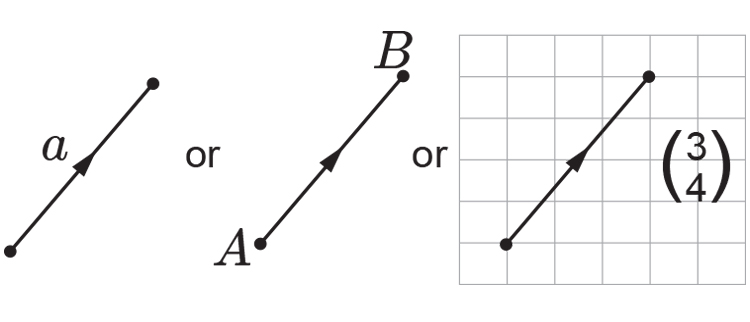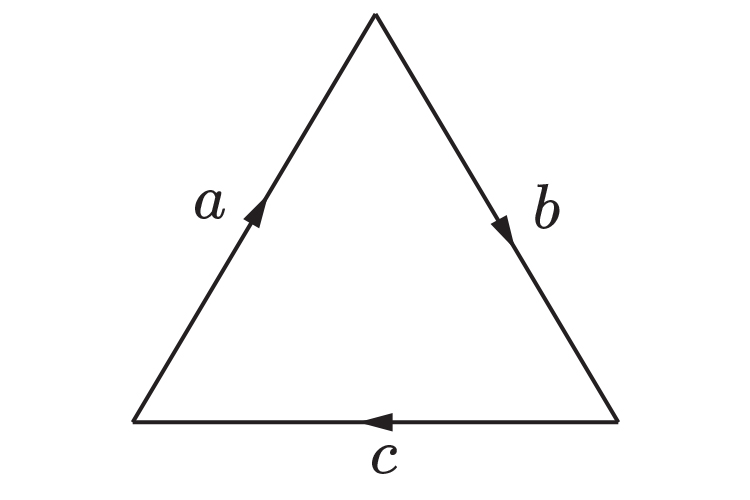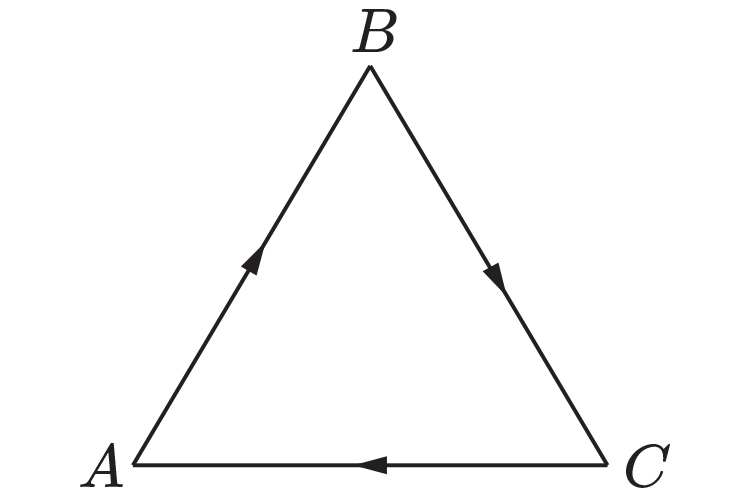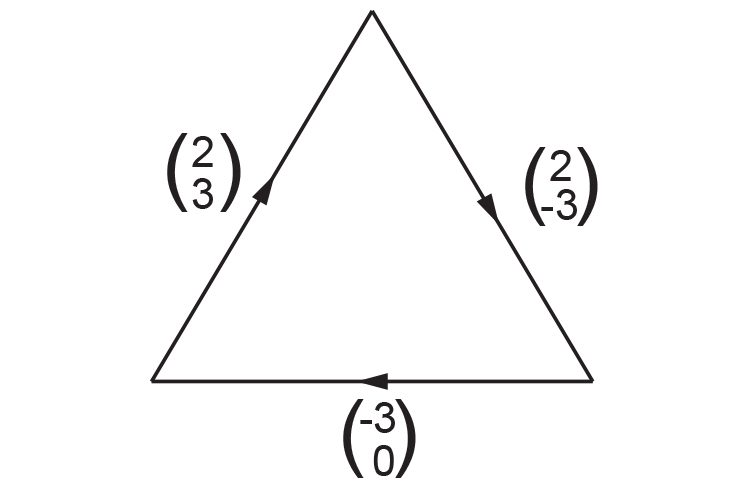# Representing vectors

You can represent vectors in 3 ways:

 a or vec (AB) or ((3),(4)) Lower case letter Capitals with an arrow above Column

As followsFurther examples

Example 1This triangle is made up of three vectors:

a,  b  and c

Example 2This triangle is made up of three vectors:

vec (AB), vec (BC)  and vec (CA)

Example 3This triangle is made up of three vectors:

((2),(3))  and ((2),(-3))  and ((-3),(0))